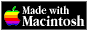THE HYPERCARD CENTER

 HyperTalk Reference
 Home » HyperTalk Reference » Functions Select a destination << Home Stack < HyperTalk Reference absannuityatanaveragecharToNumclickChunkclickH and clickVclickLineclickLocclickTextcommandKeycompoundcosdatedestinationdiskSpaceexp, exp1, exp2foundChunkfoundFieldfoundLinefoundTextheapSpacelengthln, ln1, log2maxmenusminmousemouseClickmouseH and mouseVmouseLocnumbernumToCharoffsetoptionKeyparamparamCountparamsprogramsrandomresultroundscreenRectsecondsselectedButtonselectedChunkselectedFieldselectedLineselectedLocselectedTextshiftKeysinsoundsqrtstacksstackSpacesumsystemVersiontantargettickstimetooltruncvaluewindows

# annuity

`annuity(number1, number2)`

where` number1 `represents the interest rate (expressed as a decimal) and` number2 `represents the number of periods over which you receive annuity payments.

Value returned: the total cost of an annuity now that will pay you one unit per period over the specified number of periods, or a number equal to `(1 - (1 + rate) ^ -periods) / rate`

An interest rate involves a certain percentage (expressed as a decimal) per some unit of time—usually per year. You must use the same unit of time to measure the number of periods.

For example, if you have a yearly percentage rate but your annuity pays you monthly, use `rate / 12,` and be sure to express the number of periods as months (2 years = 24 months).

## Related Topics

Version 0.7b1 (March 24, 2022)Switch to Modern View